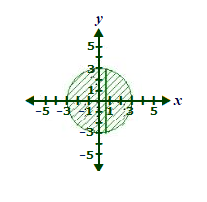# The base of a three-dimensional figure is bounded by the circle x^{2} + y^{2} = 9. Vertical...

## Question:

The base of a three-dimensional figure is bounded by the circle {eq}x^{2} + y^{2} = 9 {/eq}. Vertical cross-sections that are perpendicular to the {eq}x {/eq}-axis are rectangles with height equal to twice the width.

Algebraically, find the area of each rectangle.## Area Of A Rectangle:

The Area of a rectangle whose base is given by a function {eq}f(x) {/eq} and height is given by a function {eq}g(x) {/eq} is given by the function,

{eq}A(x)=f(x)g(x) {/eq}

The equation of the circular base is,

{eq}x^2+y^2=9\\ \Rightarrow y=\sqrt{9-x^2} {/eq}

Now considering a cross section at a distance {eq}x {/eq} from the Y-axis we will have its width as,

{eq}\text{ Width }=2y=2\sqrt{9-x^2} {/eq}

So the corresponding height of that cross-section will be,

{eq}\text{ Height }=2\times \text{ Width }=2(2\sqrt{9-x^2})=4\sqrt{9-x^2} {/eq}

Finally, the area of the cross section will be,

{eq}\begin{align} \text{ Area } &=\text{ Width }\times \text{ Height }\\ &=\left( 2\sqrt{9-x^2}\right)\left(4\sqrt{9-x^2} \right)\\ &=8(9-x^2)\\ &=72-8x^2 \end{align} {/eq}

Thus the area of any cross-section ( rectangle ) can be found by substituting its distance {eq}x {/eq} from the Y-axis.